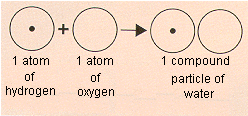John Dalton

Modern Atomic Theory (John Dalton)

Experiments with gases that first became possible at the turn of the nineteenth century led John Dalton in 1803 to propose a modern theory of the atom based on the following assumptions.

1. Matter is made up of atoms that are indivisible and indestructible.

2. All atoms of an element are identical.

3. Atoms of different elements have different weights and different chemical properties.

4. Atoms of different elements combine in simple whole numbers to form compounds.

5. Atoms cannot be created or destroyed. When a compound decomposes, the atoms are recovered unchanged.

Dalton's Law of Partial Pressures (John Dalton)

John Dalton was the first to recognize that the total pressure of a mixture of gases is the sum of the contributions of the individual components of the mixture. By convention, the part of the total pressure of a mixture that results from one component is called the partial pressure of that component. Dalton's law of partial pressures states that the total pressure of a mixture of gases is the sum of the partial pressures of the various components.

PT = P1 + P2 + P3 + ...

Dalton derived the law of partial pressures from his work on the amount of water vapor that could be absorbed by air at different temperatures. It is therefore fitting that this law is used most often to correct for the amount of water vapor picked up when a gas is collected by displacing water.

Origins of Stoichiometry (John Dalton)

John Dalton was not familiar with Richter's work when he developed his atomic theory in 1803. By 1807, however, references to this work appeared in Dalton's notebooks, and Dalton's contemporaries viewed his atomic theory as a way of explaining why compounds combine in definite proportions.

Consider water, for example. In his famous textbook, Trait lmentaire de Chimie, which was published in 1789, Lavoisier reported that water was roughly 85% oxygen and 15% hydrogen by weight. Water therefore seemed to contain 5.6 times more oxygen by weight than hydrogen. Dalton assumed that water contains one atom of hydrogen and one atom of oxygen, as shown below, and concluded that an oxygen atom must weigh 5.6 times more than a hydrogen atom. On the basis of such reasoning, Dalton constructed a table of the relative atomic weights of a handful of elements.Dalton assumed that water contains one atom of hydrogen and one atom of oxygen and concluded that the relative weight of the oxygen atom must be 5.6 times as large as the hydrogen atom.